# Multiply each element in a List by a Number in PythonLast updated: Dec 17, 2022
6 min## #Multiply each element in a list by a number in Python

To multiply each element in a list by a number:

1. Use a list comprehension to iterate over the list.
2. On each iteration, multiply the current element by the number.
3. The new list will contain the multiplication results.
main.py
```Copied!```# ✅ Multiply each element in a list by a number
import math

my_list = [2, 4, 6]

result = [item * 10 for item in my_list]
print(result)  # 👉️ [20, 40, 60]

# ------------------------------------------

# ✅ Multiply all elements in a list

my_list = [2, 4, 6]

result = math.prod(my_list)
print(result)  # 👉️ 48
``````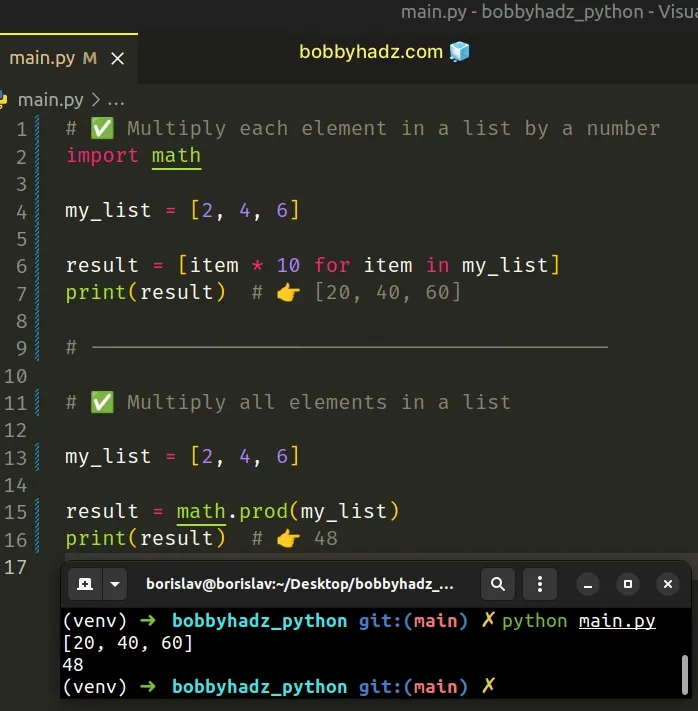We used a list comprehension to iterate over the list and multiplied each list item by `10`.

List comprehensions are used to perform some operation for every element, or select a subset of elements that meet a condition.

On each iteration, we multiply the current list item by the specified number and return the result.

Alternatively, you can use a simple for loop.

## #Multiply each element in a list by a number using a `for` loop

This is a four-step process:

1. Declare a new variable that stores an empty list.
2. Use a `for` loop to iterate over the original list.
3. On each iteration, multiply the current list item by the number.
4. Append the result to the new list.
main.py
```Copied!```my_list = [2, 4, 6]

result = []

for item in my_list:
result.append(item * 10)

print(result) # 👉️ [20, 40, 60]
``````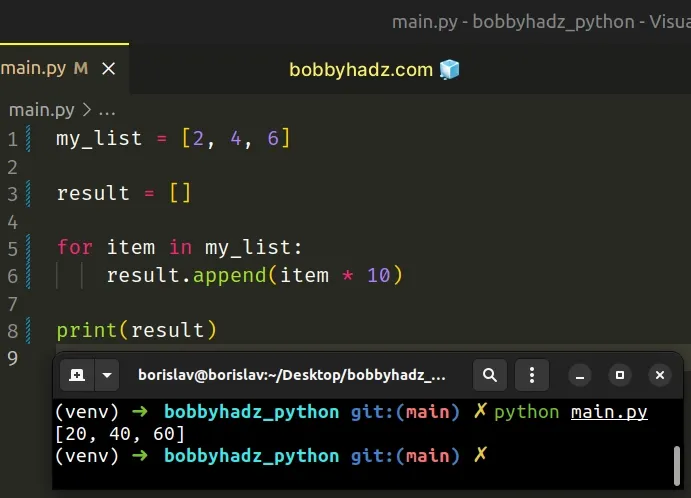The `for` loop works in a very similar way to the list comprehension, but instead of returning the list items directly, we append them to a new list.

## #Multiply each element in a list by a number using `map()`

You can also use the `map()` function to multiply each element in a list.

main.py
```Copied!```my_list = [2, 4, 6]

result = list(map(lambda item: item * 10, my_list))

print(result)  # 👉️ [20, 40, 60]
``````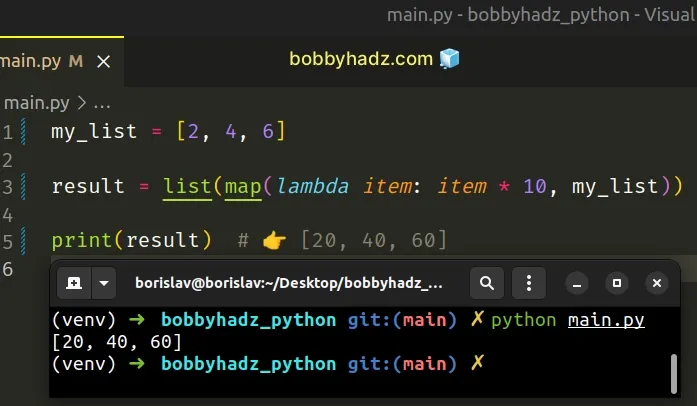The map() function takes a function and an iterable as arguments and calls the function with each item of the iterable.

The lambda function we passed to `map` gets called with each item in the list, multiplies the item by `10` and returns the result.

The last step is to use the `list()` class to convert the `map` object to a `list`.

## #Multiply each element in a list by a number using NumPy

If you work with NumPy arrays, you can directly use the multiplication operator on the array to multiply each of its elements by a number.

main.py
```Copied!```import numpy as np

arr = np.array([2, 4, 6])

result = arr * 10

print(result)  # 👉️ [20 40 60]
``````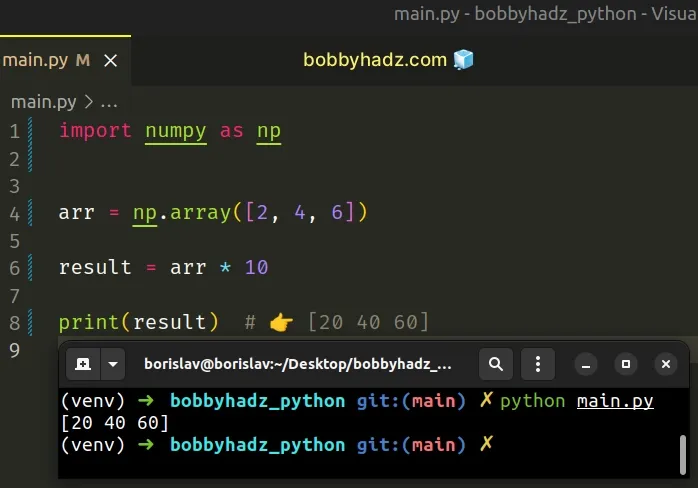Multiplying a NumPy array by a number effectively multiplies each element in the array by the specified number.

Note that this only works with NumPy arrays. If you multiply a python list by a number, it gets repeated N times.

main.py
```Copied!```print([2, 4, 6] * 2) # 👉️ [2, 4, 6, 2, 4, 6]
``````

Multiplying a Python list by N returns a new list containing the elements of the original list repeated N times.

## #Multiply all elements in a List in Python

If you need to multiply all elements in a list, use the `math.prod()` function.

The `math.prod()` method calculates the product of all the elements in the provided iterable.

main.py
```Copied!```import math

my_list = [2, 3, 5]

result = math.prod(my_list)
print(result)  # 👉️ 30
``````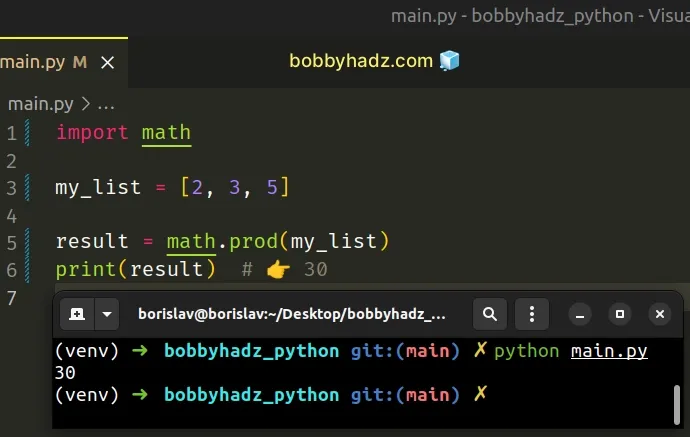Make sure to import the `math` module at the top.

We used the `math.prod` method to multiply all the elements in a list.

The math.prod method calculates the product of all the elements in the provided iterable.

main.py
```Copied!```import math

my_list = [5, 5, 5]

result = math.prod(my_list)

print(result) # 👉️ 125
``````

The method takes the following 2 arguments:

NameDescription
iterableAn iterable whose elements to calculate the product of
startThe start value for the product (defaults to `1`)

If the iterable is empty, the `start` value is returned.

Alternatively, you can use the `reduce()` function.

## #Multiply all elements in a List using `reduce()`

This is a two-step process:

1. Pass a lambda function and the list to the `reduce()` function.
2. The lambda function should take the accumulator and the current value and should return the multiplication of the two.
main.py
```Copied!```from functools import reduce

my_list = [2, 3, 5]

result = reduce(lambda x, y: x * y, my_list)
print(result) # 👉️ 30
``````

The reduce function takes the following 3 parameters:

NameDescription
functionA function that takes 2 parameters - the accumulated value and a value from the iterable.
iterableEach element in the iterable will get passed as an argument to the function.
initializerAn optional initializer value that is placed before the items of the iterable in the calculation.
The lambda function gets called with the accumulated value and the value of the current iteration and multiplies them.

If we provide a value for the `initializer` argument, it is placed before the items of the iterable in the calculation.

main.py
```Copied!```from functools import reduce

my_list = [2, 3, 5]

def do_math(acc, curr):
print(acc)  # 👉️ is 10 on first iteration
return acc * curr

result = reduce(do_math, my_list, 10)
print(result)  # 👉️ 300
``````

We passed `10` for the initializer argument, so the value of the `accumulator` will be `10` on the first iteration.

The value of the `accumulator` would get set to the first element in the iterable if we didn't pass a value for the `initializer`.

If the `iterable` is empty and the `initializer` is provided, the `initializer` is returned.

If the `initializer` is not provided and the iterable contains only `1` item, the first item is returned.

main.py
```Copied!```from functools import reduce

my_list = 

result = reduce(lambda acc, curr: acc * curr, my_list)
print(result)  # 👉️ 2
``````

## #Multiply all elements in a List using a `for` loop

You can also use a `for` loop to multiply all elements in a list.

main.py
```Copied!```my_list = [2, 3, 5]

result = 1

for item in my_list:
result = result * item

print(result) # 👉️ 30
``````

On each iteration of the `for` loop, we multiply the `result` variable by the current list item and reassign it to the result.

## #Multiply two lists element-wise in Python

To multiply two lists element-wise:

1. Use the `zip` function to get an iterable of tuples with the corresponding items.
2. Use a list comprehension to iterate over the iterable.
3. On each iteration, multiply the values in the current tuple.
main.py
```Copied!```list_1 = [1, 2, 3]
list_2 = [4, 5, 6]

# 👇️ [(1, 4), (2, 5), (3, 6)]
print(list(zip(list_1, list_2)))

result = [x * y for x, y in zip(list_1, list_2)]

print(result)  # 👉️ [4, 10, 18]
``````

The zip function iterates over several iterables in parallel and produces tuples with an item from each iterable.

main.py
```Copied!```list_1 = [1, 2, 3]
list_2 = [4, 5, 6]

# 👇️ [(1, 4), (2, 5), (3, 6)]
print(list(zip(list_1, list_2)))
``````

You can imagine that the `zip()` function iterates over the lists, taking 1 item from each.

The first tuple in the list consists of the elements in each list with an index of `0`, the second tuple consists of the elements in each list that have an index of `1`, etc.

The last step is to use a list comprehension to iterate over the `zip` object and multiply the values in each tuple.

main.py
```Copied!```list_1 = [1, 2, 3]
list_2 = [4, 5, 6]

# 👇️ [(1, 4), (2, 5), (3, 6)]
print(list(zip(list_1, list_2)))

result = [x * y for x, y in zip(list_1, list_2)]

print(result)  # 👉️ [4, 10, 18]
``````
List comprehensions are used to perform some operation for every element, or select a subset of elements that meet a condition.

On each iteration, we unpack the values from the tuple and use the multiplication `*` operator to multiply them.

main.py
```Copied!```a, b = (2, 5)

print(a * b) # 👉️ 10
``````

## #Multiplying more than two lists element-wise

You can also use this approach to multiply more than two lists element-wise.

main.py
```Copied!```list_1 = [1, 2, 3]
list_2 = [4, 5, 6]
list_3 = [7, 8, 9]

# 👇️ [(1, 4, 7), (2, 5, 8), (3, 6, 9)]
print(list(zip(list_1, list_2, list_3)))

result = [x * y * z for x, y, z in zip(list_1, list_2, list_3)]

print(result)  # 👉️ [28, 80, 162]
``````

Alternatively, you can use the `map()` function.

## #Multiply two lists element-wise using `map()`

This is a two-step process:

1. Use the `map()` function to call the `mul()` function with the two lists.
2. Use the `list()` class to convert the map object to a list.
main.py
```Copied!```from operator import mul

list_1 = [1, 2, 3]
list_2 = [4, 5, 6]

result = list(map(mul, list_1, list_2))

print(result)  # 👉️ [4, 10, 18]
``````

The map() function takes a function and an iterable as arguments and calls the function with each item of the iterable.

The `mul` function from the `operator` module is the same as `a * b`.

You can imagine that `map` calls the `mul` function with each item of the two iterables (e.g. items at index `0`, then `1`, etc).

The `map` function returns a `map` object, so we had to use the `list()` class to convert the result to a list.